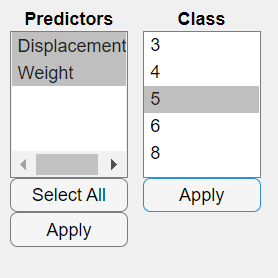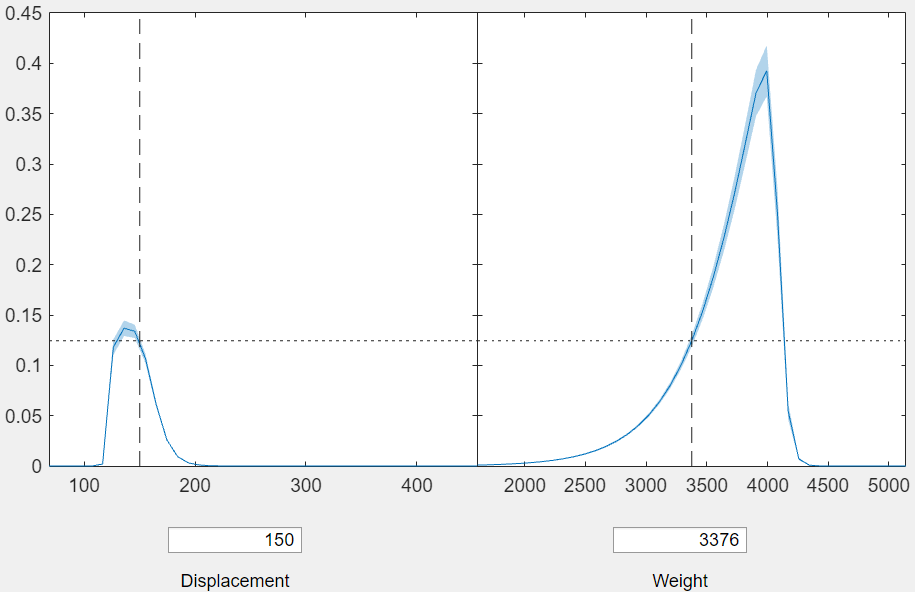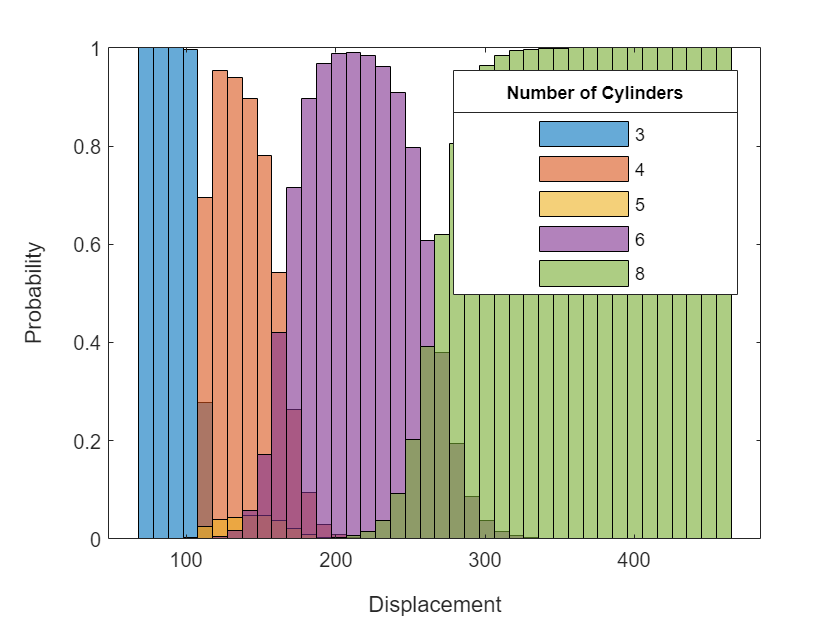# plotSlice

Plot of slices through fitted multinomial regression surface

Since R2023a

## Syntax

`` plotSlice(mdl)``
`` plotSlice(mdl,plotType)``
`` plotSlice(ax,___)``
`` plotSlice(___,Name=Value)``
``h = plotSlice(___)``

## Description

example

```` plotSlice(mdl)` generates an interactive figure containing a plot for each predictor variable. Each plot shows the fitted category probability of the first response category as a function of its corresponding predictor variable, with the other predictor variables fixed at their sample means. You can customize the figure by selecting a different response class, changing the values of the fixed predictors, and selecting a subset of predictor variables.```
```` plotSlice(mdl,plotType)` specifies the type of plot to generate: an interactive figure, a histogram, or a stacked histogram.```
```` plotSlice(ax,___)` plots on the axes specified by `ax` instead of the current axes, using any of the input argument combinations in the previous syntaxes. Note that `plotType` must be `"histogram"` or `"stackedhist"`.```

example

```` plotSlice(___,Name=Value)` specifies additional options using one or more name-value arguments. For example, you can specify the predictor variables for a histogram.```
````h = plotSlice(___)` returns a handle for the `Figure` object, `Histogram` array, or `Bar` array. Use `h` to modify the properties of the figure, histogram, or stacked histogram after you create the plot. For a list of properties, see Figure Properties, Histogram Properties, or Bar Properties.```

## Examples

collapse all

Load the `carbig` sample data set.

`load carbig`

The variables `Displacement`, `Weight`, and `Cylinders` contain data for car engine displacement, weight, and number of engine cylinders, respectively.

Fit a multinomial regression model using `Displacement` and `Weight` as predictor variables and `Cylinders` as the response data. Display the vector of response category names.

```mdl = fitmnr([Displacement,Weight],Cylinders,PredictorNames=["Displacement","Weight"]); mdl.ClassNames```
```ans = 5×1 3 4 5 6 8 ```

The output shows that the first category in the vector of response category names corresponds to cars with three engine cylinders.

Generate plots for the probability of a car having three engine cylinders as a function of each predictor variable.

`plotSlice(mdl)`By default, `plotSlice` displays the probability for the first response category in `ClassNames`. The two plots for the predictor variables share a vertical axis. In the left plot, the `Weight` predictor is fixed at `3376.5` whereas the `Displacement` predictor varies. In the right plot, `Displacement` is fixed at `261.5` whereas `Weight` varies. The probability of a car having three cylinders is small for all values of `Weight` when `Displacement` is `261.5`.

To change the response category to cars with five engine cylinders, click Class then click Select. In the dialog box, click `5` then click OK.`plotSlice` updates the plots to display the probability that a car has five engine cylinders.The right plot shows that the probability of a car having five cylinders is also small for all values of `Weight` when `Displacement` is `261.5`.

To fix `Displacement` at `150` in the right plot, enter `150` in the Displacement box.The shared vertical axis automatically rescales to accommodate larger values in the right plot. The right plot shows that when `Displacement` is fixed at `150`, the probability of a car having five engine cylinders peaks when `Weight` is approximately `4000`.

Load the `carbig` sample data set.

`load carbig`

The variables `Displacement`, `Weight`, and `Cylinders` contain data for car engine displacement, weight, and number of engine cylinders, respectively.

Fit a multinomial regression model using `Displacement` and `Weight` as predictor variables and `Cylinders` as the response data. Display the vector of response category names.

```mdl = fitmnr([Displacement,Weight],Cylinders,PredictorNames=["Displacement","Weight"]); mdl.ClassNames```
```ans = 5×1 3 4 5 6 8 ```

The output shows that the response categories correspond to cars with three, four, five, six, and eight engine cylinders.

Display the vector of predictor names.

`mdl.PredictorNames`
```ans = 2x1 cell {'Displacement'} {'Weight' } ```

The output shows that the first predictor in the vector of predictor names corresponds to `Displacement`.

Generate overlayed histograms of the estimated probabilities for each response category. By default, `plotSlice` varies the first predictor `Displacement` and fixes the value of the remaining predictor `Weight` at its training data mean.

```plotSlice(mdl,"histogram") lgd = legend; title(lgd,"Number of Cylinders")```The output shows that the probability for each response category peaks in a different interval of `Displacement`. Cars with higher engine displacement have a higher probability of having more cylinders.

Fix the value of `Displacement` at the training data mean, and then plot histograms of the response category probabilities for varying `Weight`.

```plotSlice(mdl,"histogram",PredictorToVary="Weight") lgd = legend; title(lgd,"Number of Cylinders")```The output shows that a car is most likely to have an engine with six cylinders for all values of `Weight`. Together, the plots show that the probability of a car being in each category depends on `Displacement` more than `Weight`.

## Input Arguments

collapse all

Multinomial regression model object, specified as a `MultinomialRegression` model object created with the `fitmnr` function.

Type of plot to generate, specified as one of the following options.

OptionDescription
`"interactive"` (default)Generates an interactive figure containing a plot for each predictor variable. The default plot shows the fitted category probability of the first response category as a function of its corresponding predictor variable, with the other predictor variables fixed at their sample means. To select a different response category, choose Select from the Class menu and then select a response category. To view plots corresponding to a different subset of predictor variables, choose Select from the Predictors menu and then select a subset of predictors. Alternatively, you can select the response category to plot by using the `ClassToPlot` name-value argument.
`"histogram"`Plots overlayed histograms of the probabilities for each response category. The default plot shows the change in the response categories as the first predictor varies, with the other predictor variables fixed at their sample means. To vary a different predictor variable, use the `PredictorToVary` name-value argument.
`"stackedhist"`Plots stacked histograms of the probabilities for each response category. The default plot shows the change in the response categories as the first predictor varies, with the other predictor variables fixed at their sample means. To vary a different predictor, use the `PredictorToVary` name-value argument.

Example: `"histogram"`

Data Types: `char` | `string`

Target axes, specified as an `Axes` object. You can specify `ax` only when `plotType` is `"histogram"` or `"stackedhist"`. If you do not specify the axes, then `plotSlice` uses the current axes (`gca`).

### Name-Value Arguments

Specify optional pairs of arguments as `Name1=Value1,...,NameN=ValueN`, where `Name` is the argument name and `Value` is the corresponding value. Name-value arguments must appear after other arguments, but the order of the pairs does not matter.

Example: `plotSlice(mdl,ClassToPlot="setosa")` generates an interactive plot of the `setosa` response category.

Model Data

collapse all

Response category to plot, specified as one of the response category names in `mdl.ClassNames`. The default value for `ClassToPlot` is the first entry in `mdl.ClassNames`. This argument is valid only when `plotType` is `"interactive"`.

Example: `ClassToPlot="setosa"`

Data Types: `single` | `double` | `logical` | `char` | `string` | `cell`

Predictor to vary, specified as one of the predictor variable names in `mdl.PredictorNames`. The default value for `PredictorToVary` is the first entry in `mdl.PredictorNames`. This argument is valid only when `plotType` is `"histogram"` or `"stackedhist"`.

Example: `PredictorToVary="x1"`

Data Types: `char` | `string`

Histogram Graphics

collapse all

Histogram bar color, specified as one of these values:

• `"none"` — Bars are not filled.

• `"auto"` — The histogram bar color is selected automatically (default).

• RGB triplet, hexadecimal color code, or color name — Bars are filled with the specified color.

RGB triplets and hexadecimal color codes are useful for specifying custom colors.

• An RGB triplet is a three-element row vector whose elements specify the intensities of the red, green, and blue components of the color. The intensities must be in the range `[0,1]`; for example,``` [0.4 0.6 0.7]```.

• A hexadecimal color code is a character vector or a string scalar that starts with a hash symbol (`#`) followed by three or six hexadecimal digits, which can range from `0` to `F`. The values are not case sensitive. Thus, the color codes `"#FF8800"`, `"#ff8800"`, `"#F80"`, and `"#f80"` are equivalent.

Alternatively, you can specify some common colors by name. This table lists the named color options, the equivalent RGB triplets, and hexadecimal color codes.

Color NameShort NameRGB TripletHexadecimal Color CodeAppearance
`"red"``"r"``[1 0 0]``"#FF0000"``"green"``"g"``[0 1 0]``"#00FF00"``"blue"``"b"``[0 0 1]``"#0000FF"``"cyan"` `"c"``[0 1 1]``"#00FFFF"``"magenta"``"m"``[1 0 1]``"#FF00FF"``"yellow"``"y"``[1 1 0]``"#FFFF00"``"black"``"k"``[0 0 0]``"#000000"``"white"``"w"``[1 1 1]``"#FFFFFF"`Here are the RGB triplets and hexadecimal color codes for the default colors MATLAB® uses in many types of plots.

`[0 0.4470 0.7410]``"#0072BD"``[0.8500 0.3250 0.0980]``"#D95319"``[0.9290 0.6940 0.1250]``"#EDB120"``[0.4940 0.1840 0.5560]``"#7E2F8E"``[0.4660 0.6740 0.1880]``"#77AC30"``[0.3010 0.7450 0.9330]``"#4DBEEE"``[0.6350 0.0780 0.1840]``"#A2142F"`Example: `FaceColor="g"`

Data Types: `single` | `double` | `char` | `string`

Transparency of histogram bars, specified as a scalar value between `0` and `1` inclusive. `plotSlice` uses the same transparency for all the bars of the histogram. A value of `1` means fully opaque and `0` means completely transparent (invisible).

Example: `FaceAlpha=1`

Data Types: `single` | `double`

Width of bar outlines, specified as a positive value in point units. One point equals 1/72 inch.

Example: `LineWidth=1.5`

Data Types: `single` | `double`

Note

The graphics properties for histograms listed here are only a subset. For a complete list, see Histogram Properties.

Stacked Histogram Graphics

collapse all

Outline color, specified as `"flat"`, an RGB triplet, a hexadecimal color code, a color name, or a short name. If the plot has 150 bars or fewer, the default value is `[0 0 0]`, which corresponds to black. If the plot has more than 150 adjacent bars, the default value is `"none"`.

For a custom color, specify an RGB triplet or a hexadecimal color code.

• An RGB triplet is a three-element row vector whose elements specify the intensities of the red, green, and blue components of the color. The intensities must be in the range `[0,1]`, for example, `[0.4 0.6 0.7]`.

• A hexadecimal color code is a character vector or a string scalar that starts with a hash symbol (`#`) followed by three or six hexadecimal digits, which can range from `0` to `F`. The values are not case sensitive. Therefore, the color codes `"#FF8800"`, `"#ff8800"`, `"#F80"`, and `"#f80"` are equivalent.

Alternatively, you can specify some common colors by name. This table lists the named color options, the equivalent RGB triplets, and hexadecimal color codes.

Color NameShort NameRGB TripletHexadecimal Color CodeAppearance
`"red"``"r"``[1 0 0]``"#FF0000"``"green"``"g"``[0 1 0]``"#00FF00"``"blue"``"b"``[0 0 1]``"#0000FF"``"cyan"` `"c"``[0 1 1]``"#00FFFF"``"magenta"``"m"``[1 0 1]``"#FF00FF"``"yellow"``"y"``[1 1 0]``"#FFFF00"``"black"``"k"``[0 0 0]``"#000000"``"white"``"w"``[1 1 1]``"#FFFFFF"``"none"`Not applicableNot applicableNot applicableNo color

Here are the RGB triplets and hexadecimal color codes for the default colors MATLAB uses in many types of plots.

`[0 0.4470 0.7410]``"#0072BD"``[0.8500 0.3250 0.0980]``"#D95319"``[0.9290 0.6940 0.1250]``"#EDB120"``[0.4940 0.1840 0.5560]``"#7E2F8E"``[0.4660 0.6740 0.1880]``"#77AC30"``[0.3010 0.7450 0.9330]``"#4DBEEE"``[0.6350 0.0780 0.1840]``"#A2142F"`Example: `EdgeColor="red"`

Example: `EdgeColor=[0.6350 0.0780 0.1840]`

Example: `EdgeColor="#D2F9A7"`

Data Types: `single` | `double` | `char` | `string`

Line style, specified as one of the options listed in this table.

Line StyleDescriptionResulting Line
`"-"`Solid line`"--"`Dashed line`":"`Dotted line`"-."`Dash-dotted line`"none"`No lineNo line

Example: `LineStyle="--"`

Data Types: `char` | `string`

Note

The graphics properties for stacked histograms listed here are only a subset. For a complete list, see Bar Properties.

## Output Arguments

collapse all

Handle for the plotted objects, returned as a `Figure` object, `Histogram` array, or `Bar` array.

• When the input argument `plotType` is `"interactive"`, `plotSlice` returns `h` as a `Figure` object. For more information about `Figure` properties, see Figure Properties.

• When `plotType` is `"histogram"`, `plotSlice` returns `h` as a `Histogram` array with entries for each response variable category. For more information about `Histogram` properties, see Histogram Properties.

• When `plotType` is `"stackedhist"`, `plotSlice` returns `h` as a `Bar` array with entries for each response variable category. For more information about `Bar` properties, see Bar Properties.

## Version History

Introduced in R2023a